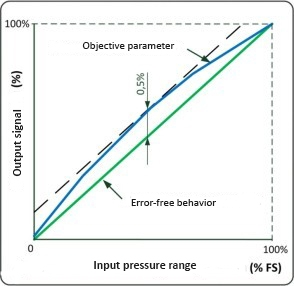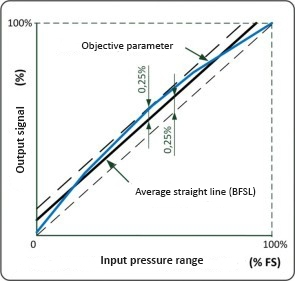What is the difference between the nonlinearity calculated by TPM method and BFSL method?

The basic accuracy specified by manufacturers of pressure and level transmitters in their datasheets includes nonlinearity, hysteresis and repeatability. Very often, next to nonlinearity data you see "BFSL" and / or "TPM". These are two different approaches to calculating nonlinearity.

TPM – is the Terminal Point Method, see fig. 1.

BFSL – is the Best Fit Straight Line method, see fig. 2

Applied, the methods provide differing nonlinearity values. As a rule, BFSL gives values twice as low as those given by TPM. So what is the difference between these methods, and how do you compare the accuracy of pressure transmitters made by different manufacturers?

 TPM This method implies connecting end points of the real characteristic with a line ( green line on fig. 1). This line and maximum deviation of the real characteristic are the variables used to calculate maximun error. In figure 1 it is 0.5%.Figure 1 (TPM) BFSL The Best Fit Straight Line method implies determining the accuracy relative to the averaged line between the extreme deviations of the real characteristic (black line). In Figure 2, the maximum error is 0.25%. As a result, we have the maximum error twice as small as that calculated with the help of TPM.Figure 2 (BFSL)

Very often, manufacturers mark the nonlinearity value as "BFSL", referring to IEC 61298-2. If there is no mark there, most likely, the value was calculated through TPM.

Comparing the accuracy of transmitters from different manufacturers, it is important to make sure that in both cases the value was calculated through the same method. Thus, you will avoid errors in comparing characteristics of pressure and level transmitters.